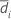# Methods and formulas for Test mean - reference mean for Equivalence Test for a 2x2 Crossover Design

The following methods and formulas are used for testing the difference between the test mean and the reference mean.

## Difference, standard error, and within-subjects standard deviation

### Difference

The difference, D, for the test of equivalence is given by the following:

### SE

The standard error for the difference is given by the following: where Sp is the pooled standard deviation, which is given by the following:

### Within-subject standard deviation

The within-subject standard deviation, Sw, is given by the following:

### Notation

TermDescriptionSample mean for sequence i (for more information, go to Methods and formulas for common concepts used in Equivalence Test for a 2x2 Crossover Design)
niNumber of participants in sequence i
SiSample standard deviation offor sequence i

## Confidence interval

### 100(1-α)% CI

By default, Minitab uses the following formula to calculate the 100(1 – α)% confidence interval (CI) for equivalence:

CI = [min(C, Dl), max(C, Du)]

where:

### 100(1-2α)% CI

If you select the option to use the 100(1 – 2α)% CI, then the CI is given by the following formula:

CI = [Dl, Du]

### One-sided intervals

For a hypotheses of Test mean > reference mean or Test mean - reference mean > lower limit, the 100(1 – α)% lower bound is equal to DL.

For a hypothesis of Test mean < reference mean or Test mean - reference mean < upper limit, the 100(1 – α)% upper bound is equal to DU.

### Notation

TermDescription
DDifference between the test mean and the reference mean
SEStandard error
δ1Lower equivalence limit
δ2Upper equivalence limit
vDegrees of freedom
αThe significance level for the test (alpha)
t1-α, vUpper 1 – α critical value for a t-distribution with v degrees of freedom

## T-values

Let t1 be the t-value for the hypothesis,, and let t2 be the t-value for the hypothesis,, whereis the difference between the mean of the test population and the mean of the reference population. By default, the t-values are calculated as follows:

For a hypothesis of Test mean > reference mean, δ1 = 0.

For a hypothesis of Test mean < reference mean, δ 2 = 0.

### Notation

TermDescription
DDifference between the sample test mean and the sample reference mean
SEStandard error of the difference
δ1Lower equivalence limit
δ2Upper equivalence limit

## P-values

The probability, PH0, for each null hypothesis (H0) is given by the following:
H0 P-Value### Notation

TermDescriptionUnknown difference between the mean of the test population and the mean of the reference population
δ1Lower equivalence limit
δ2Upper equivalence limit
vDegrees of freedom
Tt-distribution with v degrees of freedom
t1 t-value for the hypothesist2 t-value for the hypothesis###### Note

For information on how the t-values are calculated, see the section on t-values.

By using this site you agree to the use of cookies for analytics and personalized content.  Read our policy